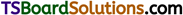# TS Inter 1st Year Chemistry Notes Chapter 1 Atomic Structure

Here students can locate TS Inter 1st Year Chemistry Notes 1st Lesson Atomic Structure to prepare for their exam.

## TS Inter 1st Year Chemistry Notes 1st Lesson Atomic Structure

→ The radiation which is made up of electrical and magnetic fields acting mutually perpendicular to each other is called Electro-magnetic radiation.

→ The number of waves that pass a point in the path of propagation of wave per second is called frequency. It is denoted by υ.

→ The number of waves present in a distance of 1 cm is called wave number. It is the reciprocal of wavelength (i.e.,) υ̅ = 1/λ.

→ According to Planck’s quantum theory emission or absorption of energy takes place in the form of small packets called Quanta. The energy of each quantum of radiation is given by E = hυ.

→ According to Einstein, both emission and absorption of radiation takes place in the form of photons. A photon is a wave particle which has no mass but has energy. The energy of a photon is given by E = hυ.

→ The emission spectrum of hydrogen consists of Lyman series, Balmer series, Paschen series, Bracket series and Pfund series.

→ According to Bohr, electrons revolve around the nucleus in certain definite circular paths, called orbits or energy levels or shells. They are denoted by the letter n.

→ Principal quantum number describes the size of the orbit and energy of the electron.

→ Azimuthal quantum number denotes the shape of the orbital.

→ Magnetic quantum number denotes the special orientation of the orbital.

→ Spin quantum number describes the direction of rotation of the revolving electron.
Its value is + 1/2 for a clockwise electron and – 1/2 for an electron revolving in the anti-clockwise direction.

→ Wave nature of electron was proposed by de Broglie.→ According to Heisenberg’s uncertainty principle, it is not possible to determine the position and the velocity of a moving particle like electron, simultaneously and accurately.

→ Ψ is known as wave function and Ψ2 is known as probability function.

→ Orbital is the space around the nucleus where the probability of finding the electron is maximum.

→ Orbitals of equal energy are called degenerate orbitals.

→ Arrangement of electrons in space around the nucleus in an atom is known as electronic configuration.

→ According to Pauli’s exclusion principle, an orbital can hold a maximum of two electrons only and a shell can hold a maximum of 2n electrons.

→ Atoms or ions with paired up electron spins * are diamagnetic and with unpaired electron spins are paramagnetic.

→ Atoms or ions with the same electronic configuration are said to be iso-electronic.

→ The wave number u of the spectral lines is given by Rydberg expression,
υ̅ = $$\frac{1}{\lambda}$$ = RH$$\left(\frac{1}{n_1^2}-\frac{1}{n_2^2}\right)$$

→ Value of Rydberg constant
(RH) = 109,677 cm-1.

→ Energy of photon, E = hυ.

→ Energy of a photoelectron,
E = hυ = hυ0 + K.E,
where υ0 = threshold frequency.

→ Mass of an electron, m = 9.1 × 10-28 g.

→ Charge of an electron, e =-4.802 × 10-10 e.s.u.(En) = $$-\frac{13.6}{n^2}$$eV
→ Value of Rydberg constant (R) = $$\frac{2 \pi^2 \mathrm{me}^4}{\mathrm{ch}^3}$$
→ de Broglie wavelength, λ = $$\frac{\mathrm{h}}{\mathrm{mV}}$$.